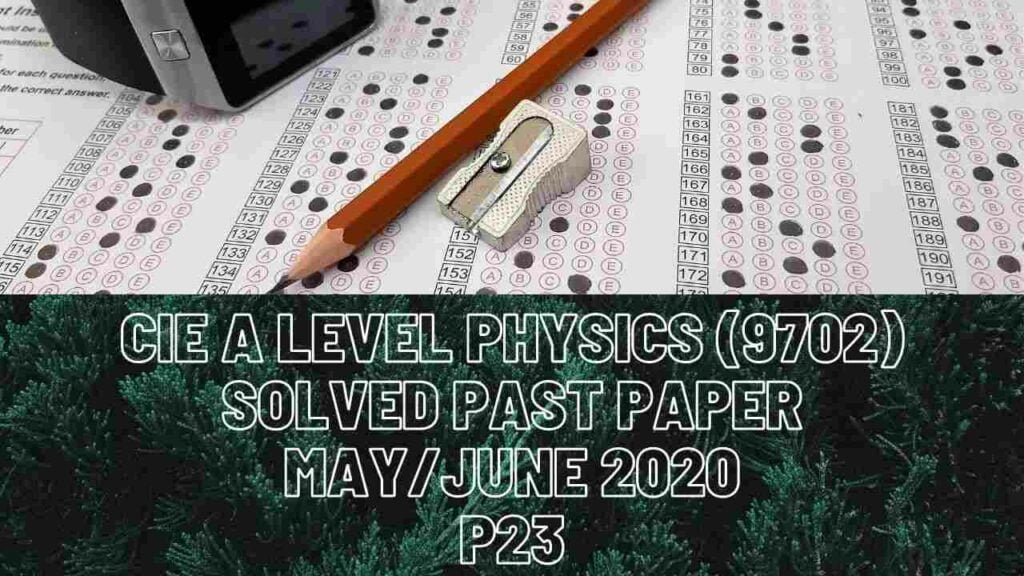A Level Physics### CIE A Level Physics Solved Past Paper May/June 2020 P23

2 aiii) Horizontal component of tension is Tcos(28). At t=2.0s, the skier is moving with acceleration which means is forward i.e tension’s horizontal component is greater than the resistive force on him that acts towards his left. At t=2.0s, acceleration is 0.8m/s^2 and his mass is 89kg. Resultant force=mass*acceleration where resultant force on the skier is difference between tension’s horizontal component and resistive force, R.

iv) At this instant, the skier is vertically at a fixed position but is moving horizontally. This means that for vertical part, he is at equilibrium, and there is no net force on him. Tension’s vertical component=his weight.

3 bi) About point A, there are 3 forces (W, W & 19N) that will cause clockwise moment and 1 force (22N) that causes anti-clockwise moment.

ii) Since the rod is at equilibrium and at rest, all upward forces must be equal to all downward forces. There are 3 forces in total 47N that act downwards and just 1 force of magnitude 22N that acts upwards so force at A must also act upwards to balance the forces.

ciii) Work done for extension of cord or elastic potential energy can be calculated by using area under graph of force versus extension graph.

4c) Phase difference of progressive wave= (distance between adjacent peaks of those 2 waves/wavelength)*360 OR (time difference on x-axis between adjacent peaks of 2 waves/time period)*360

d) Resultant displacement is sum of displacements of individual wave and that’s what law of superposition is. At 0.75s, displacement of wave Y is 4 micrometre and that of wave Z is -1 micrometre thus resultant displacement is 4+(-1)=3 micrometre

6c) When quantity radius or area doubles, it means the quantity which is inversely proportional to it decreases by 4 times.

7 ai) Electric force has a direction vertically downwards because of attraction between beta- particle and less negatively charged plate as compared to -450V.